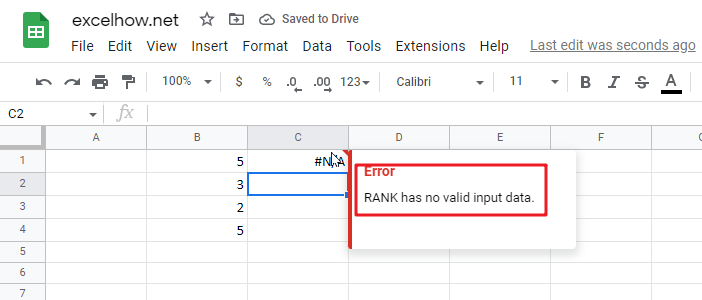## Rank Data with Multiple Criteria in google sheets

If you are an enthusiastic Google Sheets user, then you may have an urgent task to rank a specified datelist based on given multiple criteria; you can easily perform this task manually, which is also feasible, but it only works for ranking a small amount of data. However, when there are multiple pieces of data to be processed, it becomes a cumbersome and time-consuming task to sort the list of data based on multiple conditions by manually.

But don’t worry, because this article will show you how to rank with multiple criteria in google sheets.

RANK Data with Multiple Criteria

If you want to rank the specified data by just one criteria, you can use the RANK function to easily rank the values of the range of cells in google sheets. Suppose you want to rank the data of a range of cells in a worksheet with multiple criteria. So how do you accomplish that task? You need to create a new complex formula by using the SUMPRODUCT function. For example, when you want to sort the list of data in the range of cells A1:B4 by using two criteria or conditions, simply use the following formula.

`=1+SUMPRODUCT((\$A\$1:\$A\$4=A1)*(\$B\$1:\$B\$4>B1))`

Next, you need to type this formula in the formula box in cell C1, then press Enter and drag the AutoFill handler to the other cells.

Let’s see the results.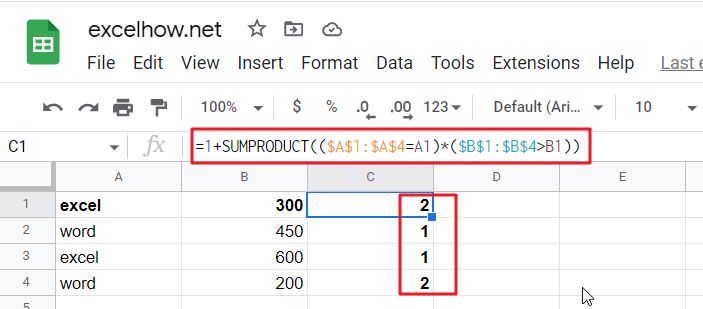### Related Functions

The Google Sheets SUMPRODUCT function multiplies corresponding components in the given one or more arrays or ranges, and returns the sum of those products.The syntax of the SUMPRODUCT function is as below:= SUMPRODUCT (array1,[array2],…)…
The Google Sheets Rank function is used to return the ranking of a given number from a list. If there are duplicate numbers in the list, then the numbers will be in the same rank.The syntax of the Google Sheets Rank function is explained as follows:The syntax of the Google Sheets Rank function is explained as follows:= RANK (number,reference ,[order])…

## Sort Dynamic Data in google sheets

This article will talk about how to sort the dynamic data in google sheets automatically. How to use RANK formula to sort the data of a given dynamic range in google sheets.

## Sort Dynamic Data

suppose you have a list of data in cell range A1:B5, and you want to sort the dynamic data in the range, you can refer to the following steps:

Step 1: You need to insert a new column before the first column of your data. Enter one name in the first cell of the new column.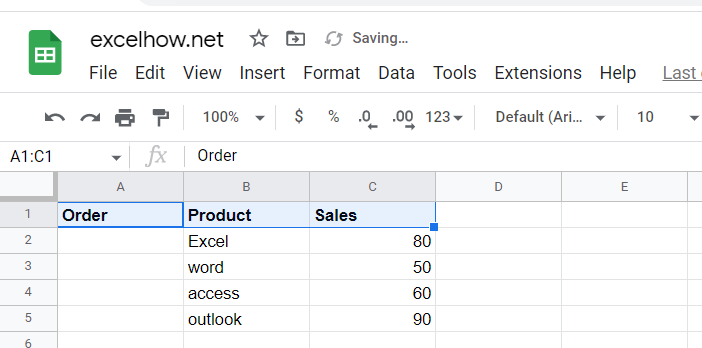Step 2: Type the following formula in cell A2, which will sort the sales values in the Sales column. Then press Enter on your keyboard and drag the AutoFill Handle over to the other cells A3:A5.

`=RANK(C2,C\$2:C\$5)`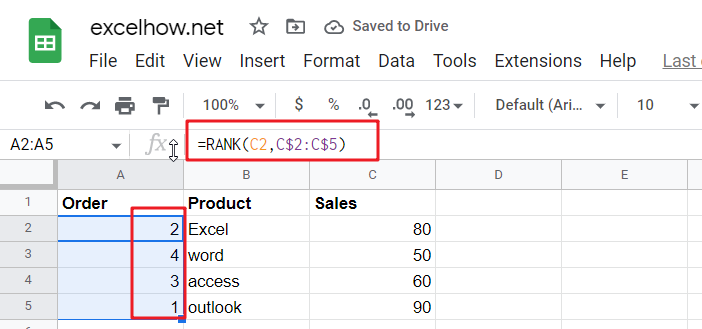Step 3: Select a new column, e.g. Column E, and enter one column name in the first cell. Select the second of the new columns adjacent to column E and enter the column name as Products. Then select the third column next to column F and enter the column name as Sales in cell G1.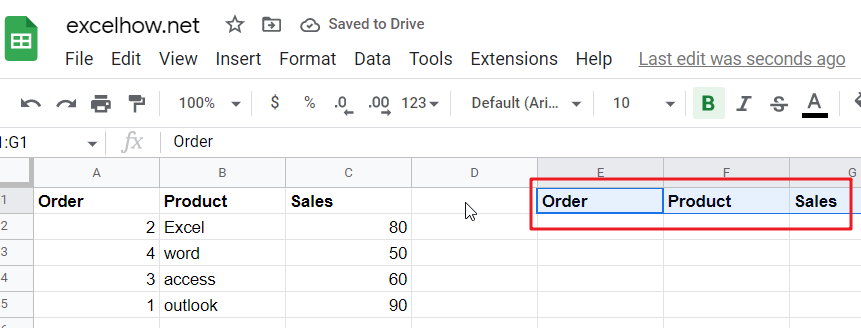Step 4: Insert the sequence number in the range of cells E2:E5.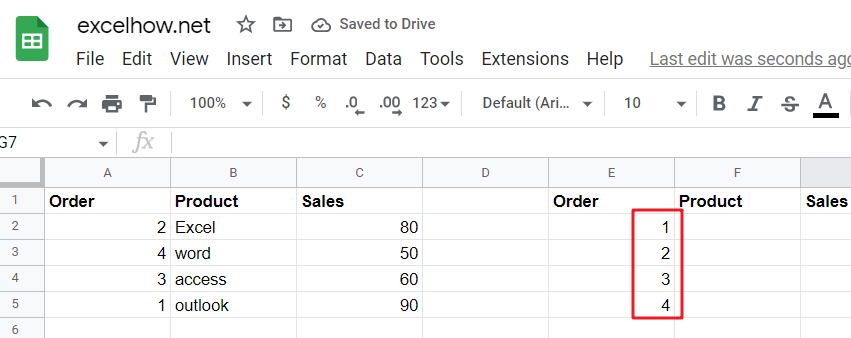Step5:  Type the following VLOOKUP formula to extract both product names and sales values in the formula box of cell F2 and press Enter. Then drag the AutoFill handle down to the other cells F3:F5 and G2:G5.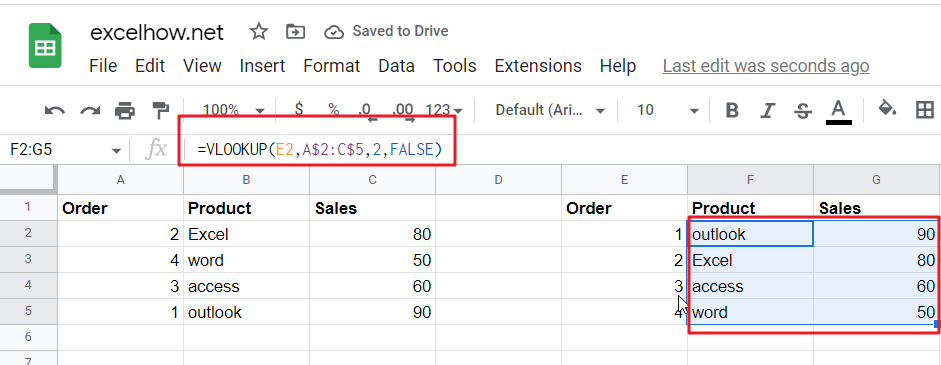Step6: You can try to change a value in the Sales column so that you can check if the product orders will be automatically sorted in the new data list E1:G5.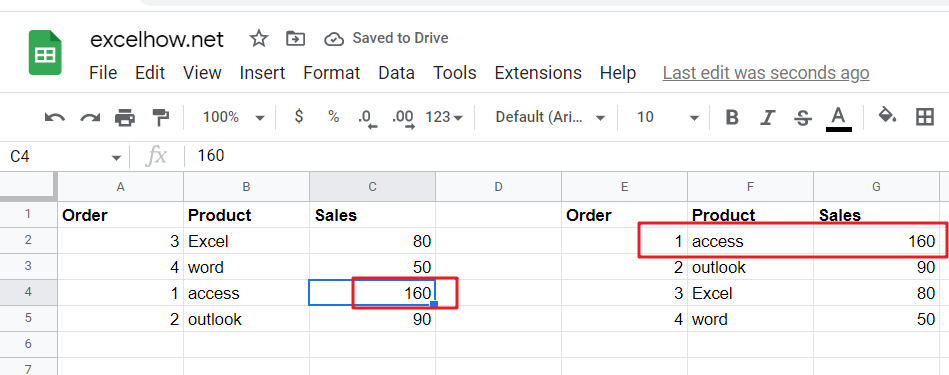### Related Functions

The Google Sheets Rank function is used to return the ranking of a given number from a list. If there are duplicate numbers in the list, then the numbers will be in the same rank.The syntax of the Google Sheets Rank function is explained as follows:The syntax of the Google Sheets Rank function is explained as follows:= RANK (number,reference ,[order])…
The Google Sheets VLOOKUP function lookup a value in the first column of the table and return the value in the same row based on index_num position.The syntax of the VLOOKUP function is as below:= VLOOKUP (lookup_value, table_array, column_index_num,[range_lookup])….

## Reverse Rank Order in google sheets

This article will talk about how to rank the given data in reverse order in google sheets. You can use Google sheets RANK function to rank the data. By default, the largest value in the data table will be ranked as 1. So how do we invert this ranking order? How do we make the smallest value ranked as 1? So how do we achieve this requirement?

## Reverse Rank Order

Suppose you have a list of data and you want to sort these data and later reverse the sort order on them? If you do the ranking by manually, it is definitely not a good way to handle it. The best way is to use the RANK function to sort the data. To reverse the sort order, you just need to add a new parameter to the RANK function. The formula is as follows.

`=RANK(B1,\$B\$1:\$B\$5,1)`

As you can see from the above example, the sorting result has been reversed.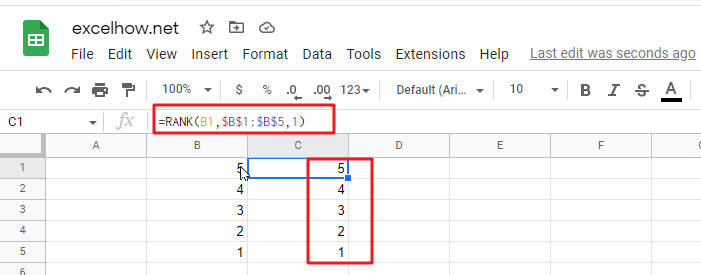### Related Functions

The Google Sheets Rank function is used to return the ranking of a given number from a list. If there are duplicate numbers in the list, then the numbers will be in the same rank.The syntax of the Google Sheets Rank function is explained as follows:The syntax of the Google Sheets Rank function is explained as follows:= RANK (number,reference ,[order])…

## Rank Numbers without Repetitive Ranks in google sheets

This article will guide you how to rank numbers in a range with unique ranking in google sheets. How to use the Rank function in google sheets to get a unique ranking for ranges with duplicate values.

## Ranking without Repetitive Ranks

Suppose you try to use the RANK function to rank values in a range of cells that have two or more duplicate values. And the RANK function will return the same ranking for duplicate values in the cell range.

For example, if you have a list of data containing values in column A (10, 12, 12, 14, 16), the RANK function will return a list of ranked values in another column as `5, 4, 4, 2, 1`. The returned result may not be the one that you expect. Because you maybe expect the result as` 5, 4, 3, 2, 1`. At this time you can use the RANK function and in combination with COUNTIF function to build a new complex google sheet formula to return a unique ranking value.

The formula is as follows：

`=RANK(A2,\$A\$2:\$A\$6,0)+COUNTIF(\$A\$2:A2,A2)-1`

You can enter the above google sheets formula in cell B2, and then press the Enter key to make the formula take effect;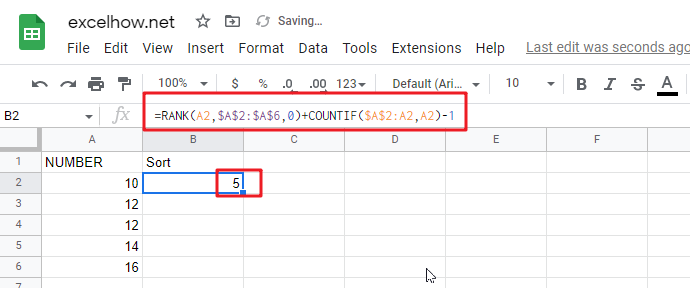Fill Handle can be dragged down until the cell B6, so that other cells can also apply the formula.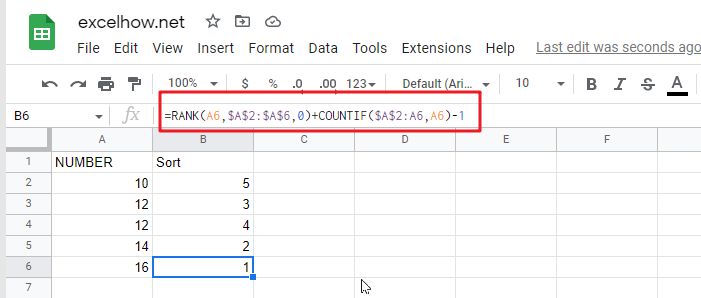From the above screenshot you can see that the unique ranking results are sorted in descending order.

If you wish the unique ranking results for the given cell range is sorted in ascending order, you can change the order type of the RANK function to `1`, the formula is changed as follows:

`=RANK(A2,\$A\$2:\$A\$6,1)+COUNTIF(\$A\$2:A2,A2)-1`

You can enter the above google sheets formula in cell C2, and then press the Enter key to make the formula take effect;

Then you can be dragged Fill Handle can be dragged down until the cell C6, so that other cells can also apply the formula.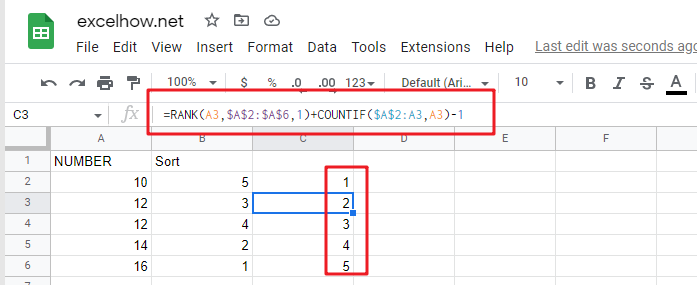### Related Functions

The Google Sheets Rank function is used to return the ranking of a given number from a list. If there are duplicate numbers in the list, then the numbers will be in the same rank.The syntax of the Google Sheets Rank function is explained as follows:The syntax of the Google Sheets Rank function is explained as follows:= RANK (number,reference ,[order])…
The google sheets COUNTIF function is used to count the number of cells in a cell range that meet a given condition.The syntax of the Google Sheets COUNTIF function is explained as follows: = COUNTIF (range, criteria)…

## Rank Numeric Values with Duplicate in Google Sheets

Suppose you have an urgent task of ranking a list with duplicate values, what would be the first approach you would think of? Is there a quick and easy way to do this task in Google Sheets? This article will talk about how to rank a given list that contains duplicate values by using the RANK formula in google Sheets.

The Rank function is a built-in function of google sheets that can be used to rank the values in a set; in other words, if the given list includes duplicate values, then the rank number will also be duplicate. See the following example.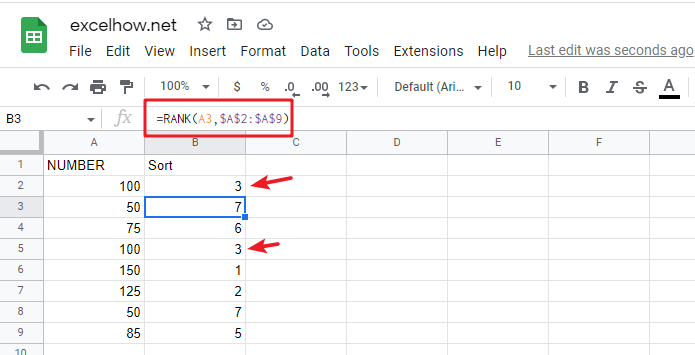From the above screenshot, you can see that the cell range A2:A9 includes 2 of the same number 100, which is ranked as 3 in the whole list, and there is no value ranked as 4 in the sorting column, because the value ranked 4 has been occupied by 3.

If we want to avoid this problem (4 should be assigned to the second 100), when we encounter a duplicate number in a set of numbers, instead of assigning it the previously used rank number, we will assign it a new rank number that follows exactly the previous rank number.

If you want to avoid the above problem of the ranked values being occupied, then you can use the following formula based on the Google Sheets RANK and COUNTIF functions：

`=RANK(A2,\$A\$2:\$A\$9)+COUNTIF(\$A\$2:A2,A2)-1`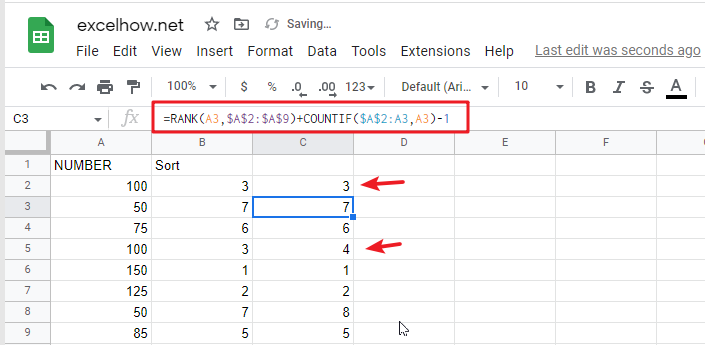Then you need to press the shortcut to allow other cells in the sorted column to apply the formula as well. CTRL+Enter

## Let’s See How This Formula Works:

As mentioned above, if you just use the Google Sheets Rank function to sort a given list of values, and as soon as it contains duplicate values, then the duplicate ranking values will be displayed in the sort results column.

When sorting a list of values, the following 2 key points need to be cleared：

• Whether the sorted result is in ascending or descending order
• Whether the sorted result can contain duplicate numbers or must be unique

### RANK EXPLANATION

You can simply think that the RANK function in google sheets is used to rank a given list of values in ascending or descending order.

Syntax:

`=RANK(number, reference, [order])`
• number – Need to determine the number of rankings
• reference– a set of numbers for ranking
• order – optional; ascending (1) or descending order (0)

You can specify whether the RANK function sorts in ascending or descending order as you need. For example, for a running race , you can use ascending order to sort, and for sales result , you can use descending order to sort. For the RANK function, the default sorting option is ascending.

### COUNTIF EXPLANATION

The COUNTIF function in google sheets is used to count the number of cells that meet a given criteria.

Syntax:

`=COUNTIF(range, criteria)`

### FORMULA EXPLANATION

=RANK(A2,\$A\$2:\$A\$9)

In this example, the numbers are arranged in a fixed range. To avoid the range being adjusted when copying the formula, so we add \$ to lock the range. This formula works well if there are no duplicate numbers, and the RANK function will assign 1 to the largest number and 2 to the second largest number according to the rule, and so on.

Unfortunately, the given list of values have duplicate values, in order to avoid duplicate ranking values, you must use the RANK function and COUNTIF function to build a new google sheets formula together.=COUNTIF(\$A\$2:A2,A2)

When copying the formula downward, the given range \$A\$2:A2 is extended, the starting cell A2 is locked, and the ending cell is the cell where the RANK function is currently working. Therefore, If the COUNTIF function encounters a duplicate value when counting, it will return the number of times that value has been repeated.

• If number only appears once, COUNTIFreturns 1 as current row is included in selected range;
• If number appears twice, COUNTIF returns 2, and so on;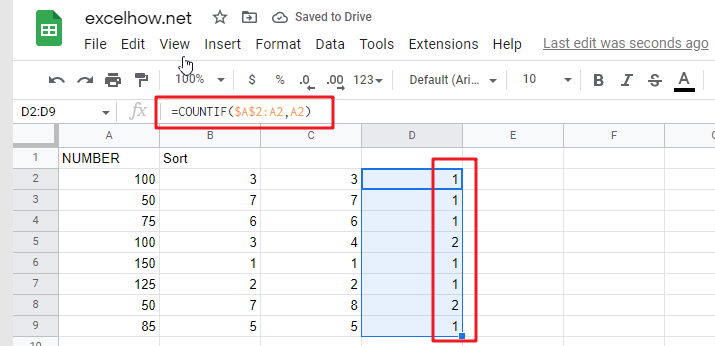=COUNTIF(\$A\$2:A2,A2)-1

Since the number appears at least once in the selected range, for example, COUNTIF(\$A\$2:A2,A2) returns 1, we use the subtraction operation “-1” to offset the value returned by COUNTIF.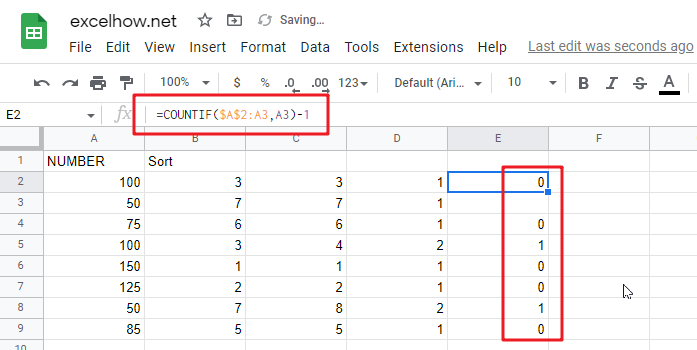### Related Functions

The Google Sheets Rank function is used to return the ranking of a given number from a list. If there are duplicate numbers in the list, then the numbers will be in the same rank.The syntax of the Google Sheets Rank function is explained as follows:The syntax of the Google Sheets Rank function is explained as follows:= RANK (number,reference ,[order])…
The google sheets COUNTIF function is used to count the number of cells in a cell range that meet a given condition.The syntax of the Google Sheets COUNTIF function is explained as follows: = COUNTIF (range, criteria)…

This article will introduce you how to use the RANK function in google sheets, and will show you a good example to better understand the usage of the RANK function.

## Google Sheets Rank Function Description

The Google Sheets Rank function is used to return the ranking of a given number from a list. If there are duplicate numbers in the list, then the numbers will be in the same rank.

The RANK function is a build-in function in Google Sheets and it is categorized as a Statistical Function.

## Google Sheets Rank Function Syntax

The syntax of the Google Sheets Rank function is explained as follows:

`= RANK (number,reference ,[order])`

Where the arguments of the RANK function are as follows:

• Number – This is a required option, the number that you would like to get ranked
• reference – This is a mandatory option, it may be an array or cell region, or a cell reference contains the specified number
• Order – This is an optional, you can specify the number of 0 or 1 to determine the type of sorting; if the value of order is 1, it means that the RANK function will be sorted in ascending order, otherwise it will be ranked in descending order.

## Google Sheets RANK Function Examples

The below examples will show you how to use Google Sheets RANK Function to get the rank of a given value within a supplied range of cells.

Example 1: If you want to get the sorting of number 2 in a given list B1:B4 in google sheets, then you can use the RANK function to do so with the following formula:

`=RANK(2,B1:B4,1)`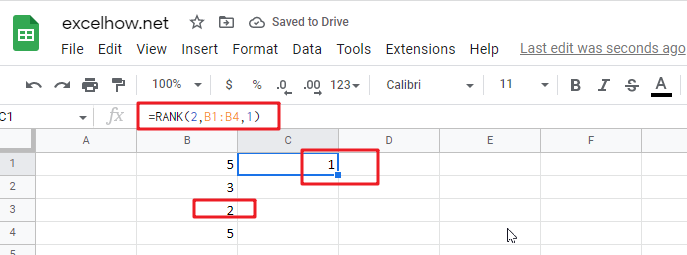Note: The number 2 must be a value that exists in B1:B4, otherwise google sheets will report the error “Rank has no valid input data“.